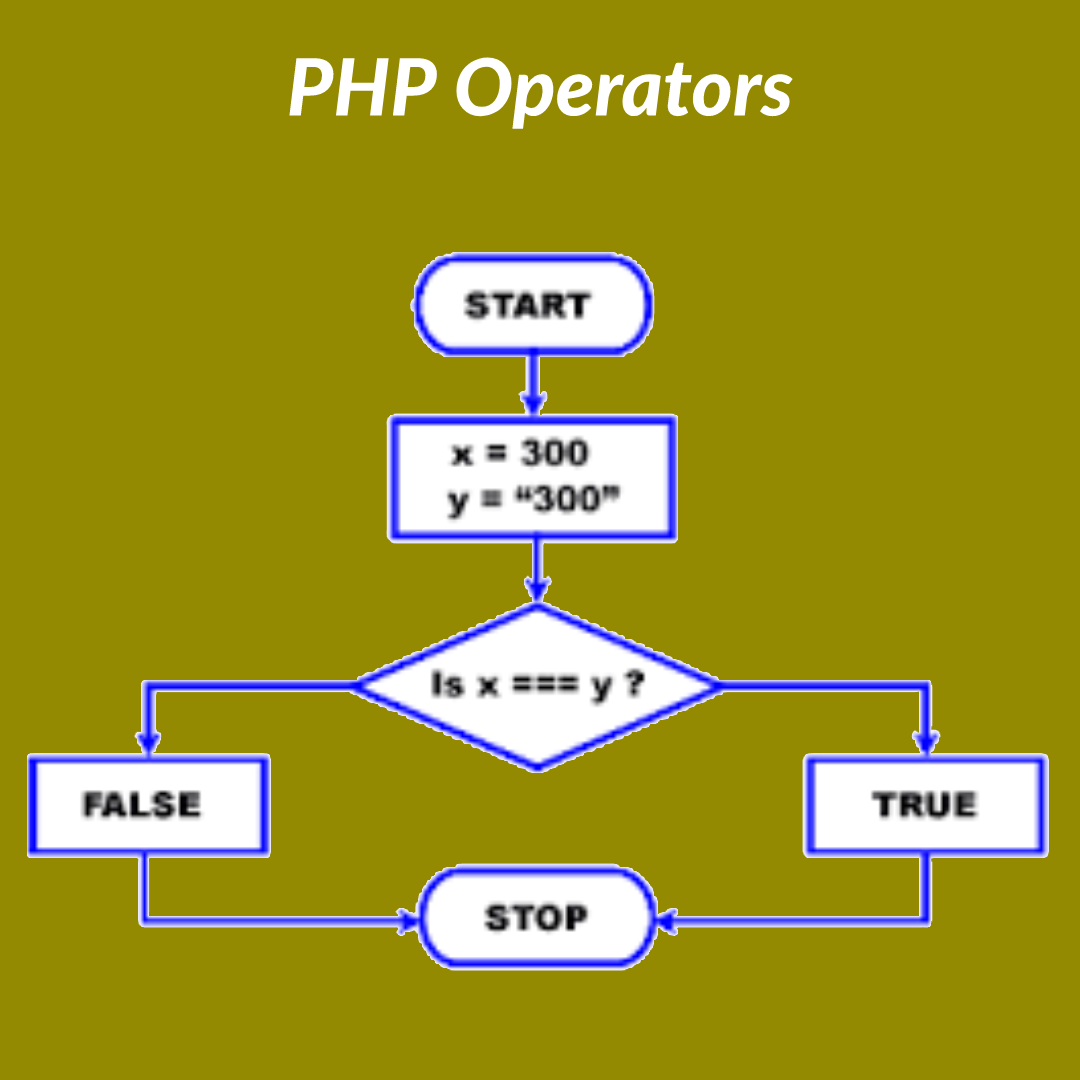# PHP OperatorsPHP Operators

PHP Operators are the characters used for mathematical, Assignment, Comparison, Logical or Concatenation Operations.

## PHP Concatenation Operators

A dot (.) is used for concatenation two or more string.

Example:
<?php
\$srr = ‘World’;
echo “Hello” . ” ” . \$srr; // it will print Hello World
?>

## PHP Mathamatical or Artithmetic Operators

the arithmetic operators are used for mathematical calculation and they are Addition, Subtraction, Multiplication, Division, Remainder of a division or Modulus, Increment and Decrements and the characters are (+), (-), (*), (/), (%), (++) and (–) the example are given below

Example:
<?php
\$i = 1+2; //now the value of veriable i is 3
\$i = 2-1; //now the value of veriable i is 1
\$i = 2*2; //now the value of veriable i is 4
\$i = 4/2; //now the value of veriable i is 2
\$i = 4%2; //now the value of veriable i is 0
\$i++; //now the value of veriable i is 1 as previous value was 0
\$i–; //now the value of veriable i is 0 as previous value was 1
?>

## PHP Assignment Operator

In php there is Assign, Increments then assigns, Decrements then assigns, Multiplies then assigns, Divides then assigns and Modulus then assigns types assignment operator and the symbols are (=), (+=), (-=), (*=), (/=) and (%=)

Example:
<?php
\$i = 1; // now the value of veriable i is 1
\$i += 2; // now the value of veriable i is 3 as previous value of i was 1 as added 2
\$i -= 1; // now the value of veriable i is 2 as previous value of i was 3 as minus 1
\$i *= 2; // now the value of veriable i is 4 as previous value of i was 2 as multyply 2
\$i /= 2; // now the value of veriable i is 2 as previous value of i was 4 as divided by 2
\$i %= 2; // now the value of veriable i is 0 as previous value of i was 2 as modulate by 2
?>

## PHP Comparison Operators

the Comparison Operators is used for compare between two value like if they are equal or not equal, grater than or less then, greater than equal or less than equal or equal with type , the example are given below and the symbols are (==) , (!), (>), (<), (>=), (<=) and (===)

Example:
<?php
\$i=1;
\$j=2;

if(\$i==1)
echo ” its a equal (==) operator “;

if(\$j!=1)
echo ” its a notequal (!=) operator “;

if(\$j>\$i)
echo ” its a grater than (>) operator “;

if(\$i<\$j)
echo ” its a less than (<) operator “;

if(\$j>=\$i)
echo ” its a grater than eual (>=) operator “;

if(\$i<=\$j)
echo ” its a less than equal (<=) operator “;

if(\$i===1)
echo ” its a equal with type (===) operator “;

?>

## PHP Logical Operators

There is 3 type of logical operator in php and they are and , or, and not. and the characters are (&&) , (||) and (!)

Example:
<?php
\$i=1;
\$j=2;

if(\$i==1 && \$j==2)
echo ” its a && operator “;

if(\$i==1 || \$j==1)
echo ” its a || operator “;

if(\$i != \$j)
echo ” its a ! operator “;

?>

I hope the post PHP Operators have given you a clear view and you have tried it by yourself.